# ML Aggarwal Class 8 Solutions for ICSE Maths Chapter 6 Operation on sets Venn Diagrams Objective Type Questions

## ML Aggarwal Class 8 Solutions for ICSE Maths Chapter 6 Operation on sets Venn Diagrams Objective Type Questions

Mental Maths
Question 1.
Fill in the blanks:
(i) If A, B are two sets, then A ∪ B = …………..
(ii) If A, B are two sets, then A ∩ B = …………..
(iii) If A, B are two sets, then A – B = …………..
(iv) A and B are disjoint sets if and only if A ∩ B = …………..
(v) A and B are overlapping sets if and only if A ∩ B = …………..
(vi) The set {x: x ϵ W, x < 3} in the roster form = …………..
(vii) If A is any set, then A ∪ ϕ = and A ∪ ξ = …………..
(viii) If ξ = {all digits in our number system] and A ={1,2, 3, 4, 5}, then A’= …………..
(ix) If A is any set and A’ is its complement, then A ∪ A’ = and A ∩ A’ = …………..
Solution: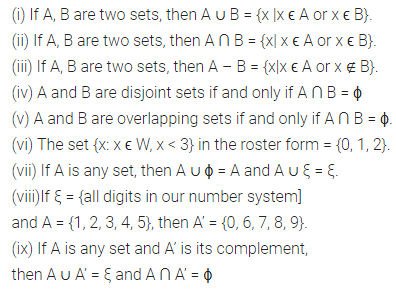Question 2.
State whether the following statements are true (T) or false (F):
(i) If ξ is the universal set and A is any set, then A’ = {x; x ϵ ξ and r ∉ A}.
(ii) If A = {0,1,2,3,4, 5} and B = {0,3, 5, 7}, then A ∩ B = B.
(in) If A= {0,1, 2,3,4, 5} and B = {0,3,5,7}, then A ∪ B=A.
(iv) If ξ = {all digits in our number system}, A = {multiples of 2} and B = {multiples of 3}, then A ∩ B = {6}.
Solution:Multiple Choice Questions
Choose the correct answer from the given four options (3 to 12):
Question 3.
If A = {x | x is a colour of rainbow} and B = {white, red, green}, then A ∩ B is
(a) B
(b) {green}
(c) {red}
(d) {green, red}
Solution: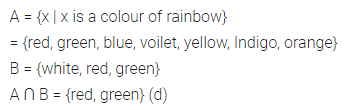Question 4.
If P = {-1, 0, 1, 2, 5} and Q = {3, 5, 7}, then P ∪ Q is
(a) {5}
(b) {-1, 0, 1, 2, 3, 7}
(c) {-1, 0, 1, 2, 3, 5, 7}
(d) none of these
Solution: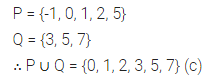Question 5.
If A and B are two sets, then A – B is defined as
(a) {x |x ϵ A or x ϵ B}
(b) {x | x ϵ A and x ϵ B}
(c) {x | x ϵ A and x ∉ B}
(d) {x | x ϵ B and x ∉ A}
Solution: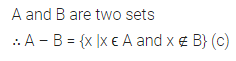Question 6.
If A is any set, then A ∪ ϕ is
(a) A
(b) ϕ
(c) 2,
(d) none of these
Solution: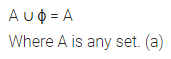Question 7.
A ∩ ξ is same as
(a) A
(b) ϕ
(c) A’
(d) ξ
Solution: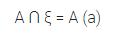Question 8.
If ξ = W and A = {x | x ϵ W and x ≤ 10}, then A’ is
(a) ϕ
(b) {x | x ϵ W and 0 ≤ x ≤ 10}
(c) {x | x ϵ W and x ≤ 10}
(d) {x | x ϵ W and x ≥ 11}
Solution:Question 9.
If ξ= {x | x ϵ W, x ≤ 12}, A – {x | x is a multiple of 3} and B = {x | x is a multiple of 4}, then A ∩ B is
(a) ϕ
(b) {0}
(c) {12}
(d) {0, 12}
Solution:Question 10.
If ξ = (all digits in our number system}, A = {x | x is prime} and B = {x | x is even}, then B – A is
(a) {4, 6, 8}
(b) {0, 4, 6, 8}
(c) {3, 5, 7}
(d) {2}
Solution: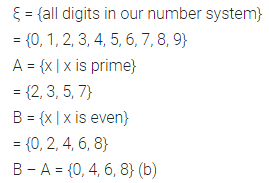Question 11.
If A and B are two sets such that n(A) = 22, n(B) = 18 and n(A ∪ B) = 35, then n(A ∩ B)
(a) 4
(b) 5
(c) 15
(d) 75
Solution: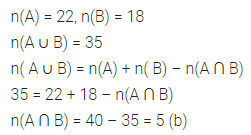Question 12.
In a town of 840 persons, 450 persons read Hindi, 300 read English and 200 read both. Then the number of person who read neither is
(a) 180
(b) 210
(c) 260
(d) 290
Solution: